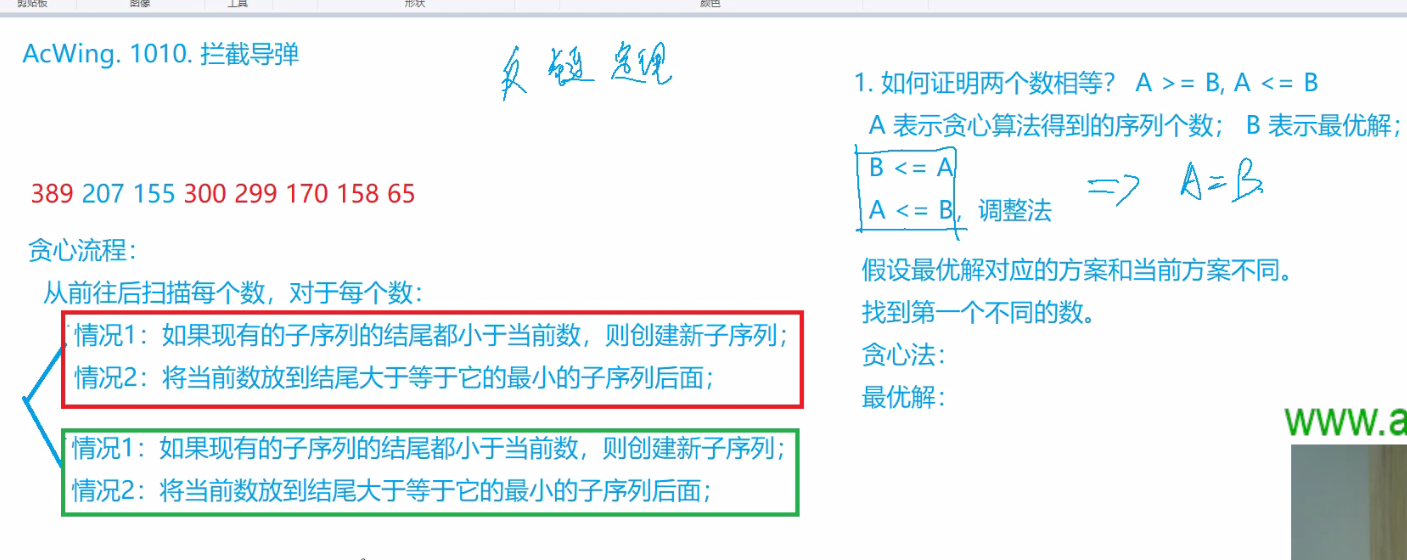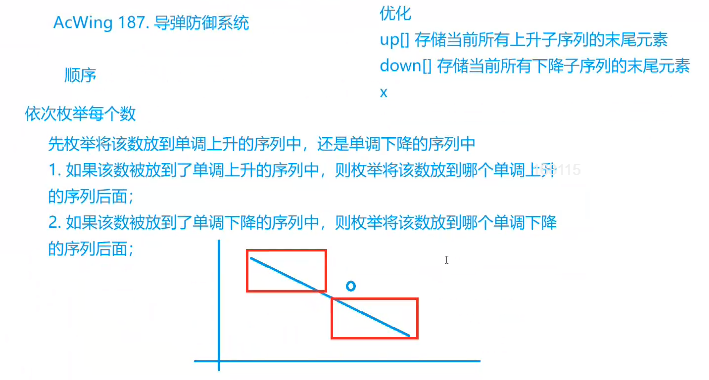从yxc老师的分析中，可以看出。我们当前数既可以存在于上升子序列数组中充当元素，也可以存在于下降子序列中充当元素。所以我们既需要带入up中去寻找其他可以，又需要去代入到down中寻找其他可能，你们就需要dfs回溯这么一个过程来求出结果。

code:

```#include<bits/stdc++.h>
using namespace std;
#pragma GCC optimize(2)
{
int x=0,f=1;char ch=getchar();
while (ch<'0'||ch>'9'){if (ch=='-') f=-1;ch=getchar();}
while (ch>='0'&&ch<='9'){x=x*10+ch-48;ch=getchar();}
return x*f;
}
int a;
int up,down;
int ans;
int n;
void dfs(int cur,int sup,int sdn)
{
if(sup+sdn-2>=ans) return;
if(cur==n+1){
ans = sup+sdn-2;
return ;
}

int k=1;
while(k<sup && up[k]>=a[cur]) k++;//其实就是贪心思想查找a[cur]的位置
int t = up[k];
up[k] = a[cur];//尝试放入
if(k<sup) dfs(cur+1,sup,sdn);//不是一个新的值
else dfs(cur+1,sup+1,sdn);//是一个新的情况
up[k] = t;//再把原来的数放回来，因为要求出所有的情况

k=1;
while(k<sdn && down[k]<=a[cur]) k++;
t = down[k];
down[k] = a[cur];
if(k<sdn) dfs(cur+1,sup,sdn);
else dfs(cur+1,sup,sdn+1);
down[k] = t;

}
int main()
{
ios_base::sync_with_stdio(0); cin.tie(0); cout.tie(0);
while(cin>>n&&n)
{
for(int i=1;i<=n;i++) cin>>a[i];
ans = n;
dfs(1,1,1);
cout<<ans<<endl;
}
}

```code：

```#include<bits/stdc++.h>
using namespace std;
#pragma GCC optimize(2)
{
int x=0,f=1;char ch=getchar();
while (ch<'0'||ch>'9'){if (ch=='-') f=-1;ch=getchar();}
while (ch>='0'&&ch<='9'){x=x*10+ch-48;ch=getchar();}
return x*f;
}
int a,up,down,n;
bool dfs(int step,int u,int su,int sd){
if(su+sd>step) return false;//上升和下降的情况大于总要求
if(u==n) return true;//如果能够包含所有的数
//将当前数放入到上升子序列的合理位置
bool flag = false;
for(int i=1;i<=su;i++)
if(up[i]<a[u]){
int t = up[i];
up[i] = a[u];
if(dfs(step,u+1,su,sd)) return true;
up[i] = t;
flag = true;
break;
}
if(!flag){
up[su+1] = a[u];
if(dfs(step,u+1,su+1,sd)) return true;
}
//将当前数放入到下降子序列的合理位置
flag = false;
for(int i=1;i<=sd;i++)
if(down[i]>a[u]){
int t = down[i];
down[i] = a[u];
if(dfs(step,u+1,su,sd)) return true;
down[i] = t;
flag = true;
break;
}
if(!flag){
down[sd+1] = a[u];
if(dfs(step,u+1,su,sd+1)) return true;
}
return false;
}
int main()
{
while(cin>>n&&n){
for(int i=0;i<n;i++) cin>>a[i];
int step = 0;
while(!dfs(step,0,0,0)) step++;
cout<<step<<endl;
}
}

```